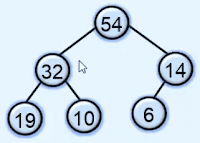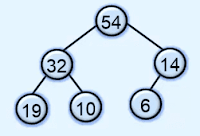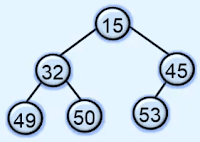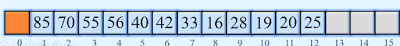# Heap in Data structures and algorithms

Heap is a Binary tree in which all levels have a maximum number of nodes except possibly the last level and in the last level all the nodes are to the left and the key in any node is greater than or equal to the keys in both children of the node.

The heap is also called a complete binary tree.here in the above tree root node has a maximum value and all the nodes have greater value in comparison of their both left and right child nodes. and in the last level, all the single nodes are on the left. so this is a heap.

## Properties of Heap

### Structure properties

All levels have a maximum number of nodes except possibly the last level
In the last level, all the nodes are to the left

### Heap order properties

Key in any node N is greater than or equal to the keys in both children of N

### Types of Heap in the data structure

Basically, the heap has two types of max heap and min-heap.

#### What is Max heap

In max heap key in any node, N is greater than or equal to the keys in both children of node N.in the above heap ever node has a greater value in comparison to their left and child node. so this is a max heap.

#### What is Min heap

In min-heap key in any node, N is smaller than or equal to the keys in both it's children.in the above example, all the nodes have smaller value in comparison to their left and child nodes. so this is a min-heap.

### Representation of Heap

as we know that the heap is a complete binary tree so it is easy to represent heap using sequential memory location.

let's say we have a heap of size n then
• we store the root in array at index 1.
• the left child of node N at index 2i.
• the right child of node n at index 2i + 1.
• Parent of node at index floor(i/2)

if the value of 2i is greater than the size of heap n then the left child of the node does not exist and if the 2i + 1 is greater than the size of heap n then the right child of the node does not exist.

Here we have a heap of size 12.to represent this heap using array first we store 85 that is a root node at index 1 and 70 and 55 which is left and right node of root node at 2i and 2i + 1 location.### Applications of Heap

Heap is used to solving the problems where the largest or smallest value has to be found. for finding the largest values we use the max heap and for finding the smallest value we use the min-heap.

We also use the heap in the selection algorithm where we need to find the kth largest element. in this problem, we build a heap a then root is deleted k times.

We use the heap to implement a priority queue because using heap the insertion and deletion become the O(log n).

We also use to sort elements using heap and this sorting algorithm is called a heap sort.

#### Program to implement Heap using python programming.

```class HeapEmptyError(Exception):
pass

class Heap:

def __init__(self, maxsize=10):
self.a = [None] * maxsize
self.n = 0        self.a = 99999
def insert(self, value):
self.n + 1        self.a[self.n] = value
self.restore_up(self.n)

def restore_up(self, i):
k = self.a[i]
iparent = i // 2
while self.a[iparent] < k:
self.a[i] = self.a[iparent]
i = iparent
iparent = i // 2        self.a[i] = k

def delete_root(self):
if self.n == 0:
raise HeapEmptyError("Heap is empty")

maxValue = self.a
self.a = self.a[self.n]
self.n -= 1        self.restore_down(1)
return maxValue

def restore_down(self, i):
k = self.a[i]
lchild = 2 * i
rchild = lchild + 1
while rchild <= self.n:
if k >= self.a[lchild] and k >= self.a[rchild]:
self.a[i] = k
return            else:
if self.a[lchild] > self.a[rchild]:
self.a[i] = self.a[lchild]
i = lchild
else:
self.a[i] = self.a[rchild]
i = rchild

lchild = 2 * i
rchild = lchild + 1
if lchild == self.n and k < self.a[lchild]:
self.a[i] = self.a[lchild]
i = lchild
self.a[i] = k

def display(self):
if self.n == 0:
print("Heap is empty")
return        print("Heap size = ", self.n)
for i in range(1, self.n + 1):
print(self.a[i], " ", end='')
print()

#####################################
h = Heap(20)
while True:
print("1. Insert")
print("2. Delete root")
print("3. Display")
print("4. Exit")
choice = int(input("Enter your choice : "))

if choice == 1:
value = int(input("Enter the value to be inserted : "))
h.insert(value)

elif choice == 2:
print("Maximum value is ", h.delete_root())
elif choice == 3:
h.display()
elif choice == 4:
break    else:
print("Wrong choice")```

1.